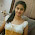## Tuesday, January 6, 2015

### Simple Circuit Schematic 100W Inverter using IC CD4047 and MOSFET IRF540

Eltronicschool. - Here is the simple circuit schematic of 100 Watt inverter using IC CD4047 as CMOS integrated circuit and MOSFET IRF540 like in figure 1 below. With this circuit schematic, we can produce more than 100 watt AC output with using 12V/ 6Ah lead acid battery as input voltage. Although that you do not expect much from this circuit. The is very simple one suitable for low grade applications.

Circuit SchematicFigure 1. Schematic 100W Inverter (Source :Circuitstoday)

Component List
1. IC CD4047
2. MOSFET IRF540
3. Resistors
4. Capacitors
5. Lead Acid Battery
6. LED
7. Capacitors
8. Transformer
9. Diode
10. All value the component see in figure 1 above

Description

Circuit schematic like in figure 1 above is Simple Circuit Schematic 100W Inverter using IC CD4047 and MOSFET IRF540. According the link source below describe that CD 4047 is a low power CMOS astable/monostable multivibrator IC. Here it is wired as an astable multivibrator producing two pulse  trains of 0.01s which are 180 degree out of phase at the pins 10 and 11 of the IC. Pin 10 is connected to the gate of Q1 and pin 11 is connected to the gate of Q2. Resistors R3 and R4 prevents the loading of the IC by the respective MOSFETs. When pin 10 is high Q1 conducts and  current flows through the upper half of the transformer primary which accounts for the positive half of the output AC voltage. When pin 11 is high Q2 conducts and  current flows through the lower half of the transformer primary in opposite direction and it accounts for the negative half of the output AC voltage.

http://www.circuitstoday.com/simple-100w-inverter-circuit

Thank you for your coming here in www.eltronicschool.com site, we hope the article above will help you to know more about your an electronic circuit design and software in this time, etc. Please comment here when you want to share and other. Thank you.

#### 1 comment:

1.Thanks for this post; Battery is the only source that assists to prevent us from the power demanding time, so choice of your battery should give at least minimum power consumption so better to buy your batteries from the battery authorized dealers
Regards,
Exide inverter battery